# Difference between revisions of "Simple Interest Formula"

## Definition

Simple Interest denotes the direct calculation of interest to the principal sum of a loan or deposit (without compounding of previous interest rate periods)

## Formula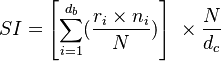$SI = \left[ \sum_{i=1}^{d_b} (\frac{r_i \times n_i}{N}) \right] \ \times \frac{N}{d_c}$

Where

•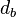$d_b$ is the number of business days in the interest period
•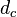$d_c$ is the number of calendar days in the interest period
•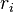$r_i$ is the interest rate applicable on business day i
•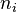$n_i$ is the number of calendar days for which rate ri applies (on most days, ni will be 1, but on a Friday it will generally be 3, and it will also be larger than 1 on the business day before a holiday).
• N is the market convention for quoting the number of days in the year.